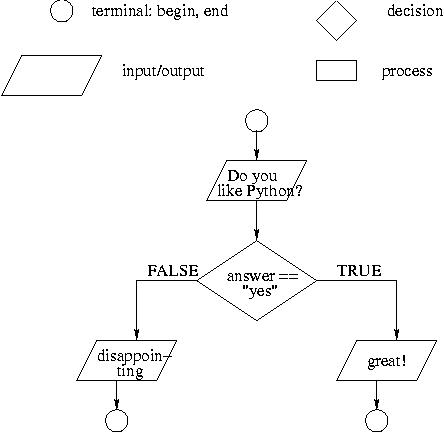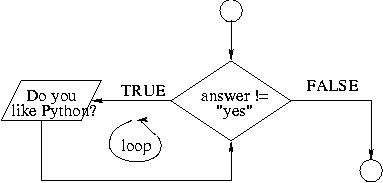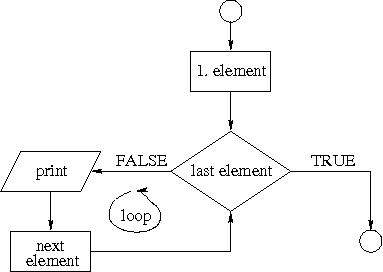#### 1) Flowcharts: if#### 2) While#!/usr/bin/env python
#
# while statement
#

while answer != "yes":
answer = raw_input("Do you like Python? ")
if answer == "yes":
print "That is great!"
else:
print "That is not the right answer! Try again."

Here is an alternative version that does the same:

#!/usr/bin/env python
#
# while statement
#
answer = raw_input("Do you like Python? ")
while answer != "yes":
print "That is not the right answer! Try again."
answer = raw_input("Do you like Python? ")
else:
print "That is great!"

#### Exercises

2.1) Modify the program from above so that it asks users to "guess the lucky number". If the correct number is guessed the program stops, otherwise it continues forever.

2.2) Modify the program so that it asks users whether they want to guess again each time. Use two variables, number for the number and answer for the answer to the question whether they want to continue guessing. The program stops if the user guesses the correct number or answers "no". (In other words, the program continues as long as a user has not answered "no" and has not guessed the correct number.)

#### 3) Modifications of a while loop

3.1) A counter: Write a program that asks five times to guess the lucky number. Use a while loop and a counter, such as

counter = 1
while counter <= 5:
print "Type in the", counter, "number"
counter = counter +1

The program asks for five guesses (no matter whether the correct number was guessed or not). If the correct number is guessed, the program outputs "Good guess!", otherwise it outputs "Try again!". After the fifth guess it stops and prints "Game over."

3.2) break: In the previous example, insert "break" after the "Good guess!" print statement. "break" will terminate the while loop so that users do not have to continue guessing after they found the number. If the user does not guess the number at all print "Sorry but that was not very successful" (use "else" for this).

3.3) Counting hits: Modify the program again. This time the program continues even after the correct number was guessed but it counts how often the correct number was guessed. You'll need two counters: one for the while loop and another one for the number of correct guesses. After the while loop is finished, use an if statement to print either "You guessed the number ... times" or "The number was not guessed at all".

Note: This strategy is used in search engines: search engines display each match but keep searching until they are through with the document collection.

#### 4) ForOther programming languages provide "for" as an alternative for while loops with counters. Python's "for" is more commonly used in connection with lists (see next week). But the following code shows how Python's "for" can be used to count from 0 to a specific number.

#!/usr/bin/env python
#
# for statement
#
for counter in range(10):
print counter

#### Exercises

4.1) Modify the counter program from above using a for loop so that it asks the user for five guesses and then stops. Use "break" to terminate the for loop as soon as the correct number is guessed.

4.2) Optional exercise: print all multiples of 13 that are smaller than 100. Use the range function in the following manner: range(start, end, step) where "start" is the starting value of the counter, "end" is the end value and "step" is the amount by which the counter is increased each time.